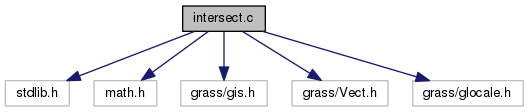GRASS Programmer's Manual  6.5.svn(2014)-r66266
vector/Vlib/intersect.c File Reference
`#include <stdlib.h>`
`#include <math.h>`
`#include <grass/gis.h>`
`#include <grass/Vect.h>`
`#include <grass/glocale.h>`
Include dependency graph for vector/Vlib/intersect.c:Go to the source code of this file.

struct  CROSS

## Macros

#define D   ((ax2-ax1)*(by1-by2) - (ay2-ay1)*(bx1-bx2))

#define D1   ((bx1-ax1)*(by1-by2) - (by1-ay1)*(bx1-bx2))

#define D2   ((ax2-ax1)*(by1-ay1) - (ay2-ay1)*(bx1-ax1))

## Functions

int Vect_segment_intersection (double ax1, double ay1, double az1, double ax2, double ay2, double az2, double bx1, double by1, double bz1, double bx2, double by2, double bz2, double *x1, double *y1, double *z1, double *x2, double *y2, double *z2, int with_z)
Check for intersect of 2 line segments. More...

int Vect_line_intersection (struct line_pnts *APoints, struct line_pnts *BPoints, struct line_pnts ***ALines, struct line_pnts ***BLines, int *nalines, int *nblines, int with_z)
Intersect 2 lines. More...

int Vect_line_check_intersection (struct line_pnts *APoints, struct line_pnts *BPoints, int with_z)
Check if 2 lines intersect. More...

int Vect_line_get_intersections (struct line_pnts *APoints, struct line_pnts *BPoints, struct line_pnts *IPoints, int with_z)
Get 2 lines intersection points. More...

## Macro Definition Documentation

 #define D   ((ax2-ax1)*(by1-by2) - (ay2-ay1)*(bx1-bx2))

Definition at line 52 of file vector/Vlib/intersect.c.

Referenced by Vect_segment_intersection().

 #define D1   ((bx1-ax1)*(by1-by2) - (by1-ay1)*(bx1-bx2))

Definition at line 53 of file vector/Vlib/intersect.c.

Referenced by Vect_segment_intersection().

 #define D2   ((ax2-ax1)*(by1-ay1) - (ay2-ay1)*(bx1-ax1))

Definition at line 54 of file vector/Vlib/intersect.c.

Referenced by Vect_segment_intersection().

## Function Documentation

 int Vect_line_check_intersection ( struct line_pnts * APoints, struct line_pnts * BPoints, int with_z )

Check if 2 lines intersect.

Points (Points->n_points == 1) are also supported.

Parameters
 [in] APoints first input line [in] BPoints second input line [in] with_z 3D, not supported (only if one or both are points)!
Returns
0 no intersection
1 intersection found

Definition at line 1091 of file vector/Vlib/intersect.c.

 int Vect_line_get_intersections ( struct line_pnts * APoints, struct line_pnts * BPoints, struct line_pnts * IPoints, int with_z )

Get 2 lines intersection points.

A wrapper around Vect_line_check_intersection() function.

Parameters
 [in] APoints first input line [in] BPoints second input line [out] IPoints output with intersection points [in] with_z 3D, not supported (only if one or both are points)!
Returns
0 no intersection
1 intersection found

Definition at line 1266 of file vector/Vlib/intersect.c.

References Vect_line_check_intersection().

 int Vect_line_intersection ( struct line_pnts * APoints, struct line_pnts * BPoints, struct line_pnts *** ALines, struct line_pnts *** BLines, int * nalines, int * nblines, int with_z )

Intersect 2 lines.

Creates array of new lines created from original A line, by intersection with B line. Points (Points->n_points == 1) are not supported.

Parameters
 [in] APoints first input line [in] BPoints second input line [out] ALines array of new lines created from original A line [out] BLines array of new lines created from original B line [out] nalines number of new lines (ALines) [out] nblines number of new lines (BLines) [in] with_z 3D, not supported!
Returns
0 no intersection
1 intersection found

Definition at line 561 of file vector/Vlib/intersect.c.

Referenced by Vect_break_lines_list(), and Vedit_bulk_labeling().

 int Vect_segment_intersection ( double ax1, double ay1, double az1, double ax2, double ay2, double az2, double bx1, double by1, double bz1, double bx2, double by2, double bz2, double * x1, double * y1, double * z1, double * x2, double * y2, double * z2, int with_z )

Check for intersect of 2 line segments.

Parameters
 [in] ax1,ay1,az1,ax2,ay2,az2 input line a [in] bx1,by1,bz1,bx2,by2,bz2 input line b [out] x1,y1,z1 intersection point1 (case 2-4) [out] x2,y2,z2 intersection point2 (case 2-4) [in] with_z use z coordinate (3D) (TODO)
Returns
0 - do not intersect,
1 - intersect at one point,
2 - partial overlap,
3 - a contains b,
4 - b contains a,
5 - identical

Definition at line 109 of file vector/Vlib/intersect.c.

References D, D1, D2, G_debug(), and G_warning().